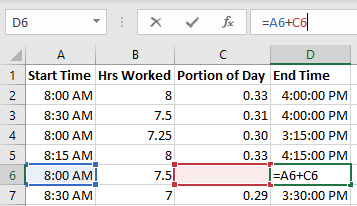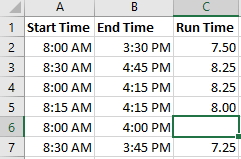Quiz

1. 1
Intermediate

2. 2
Intermediate

## Suppose cell A6 contains the time 8:00 AM, B6 the value 7.5 and D6 the formula =A6+C6 formatted as a time. Which formula for C6 returns the time 3:30 PM for D6?3. 3
Intermediate

## Suppose cells A6 and B6 contain the times 8:00 AM and 4:00 PM respectively. Which formula calculates how many hours have elapsed between these two times?